# Oppositional Teaching and Learning Based Optimization of Economical Load Dispatch Problem with Valve Point Loading Effect

Dsnm RaoChunchu Pushpa Latha Narukullapati Bharath Kumar Perumallu Mahalingam Venkatesh

Department of Electrical & Electronics Engineering, Gokaraju Rangaraju Institute of Engineering and Technology, Hyderabad 522213, Telangana, India

Department of Electrical and Electronics Engineering, G V R & S College of Engineering & Technology, Guntur 522013, Andhra Pradesh, India

Department of Electrical & Electronics Engineering, VFSTR University, Vadlamudi 522213, A.P, India

Corresponding Author Email:
2015rsee003@nitjsr.ac.in
Page:
535-540
|
DOI:
https://doi.org/10.18280/jesa.520514
11 June 2019
|
Accepted:
13 August 2019
|
Published:
13 November 2019
| Citation

OPEN ACCESS

Abstract:

Economic load dispatch (ELD) problems are traditionally solved by convex optimization techniques. However, these techniques are no longer effective if the ELD problem has a non-convex cost function. This paper aims to find a suitable meta-heuristic method to solve the ELD problem with non-convex cost function. The ELD of generators in a power system with valve point loading effect was taken as the research problem. Then, several meta-heuristic optimization techniques were compared in their abilities to find the global optimal solution, namely, the lambda iteration method, the teaching and learning based optimization (TLBO) and the oppositional teaching and learning based optimization (OTLBO). The optimization techniques were thoroughly compared through demonstrations on 6, 10, and 14 units test systems. The results show that the OTLBO outperformed the other algorithms in terms of the global optimal solution. Thus, our research confirms the feasibility and effectiveness of the OTLBO for ELD problems with valve point loading effect.

Keywords:

economic load dispatch (ELD), cost function, oppositional teaching and learning based optimization (OTLBO), valve point loading effect

1. Introduction

Nowadays, the electric energy market became more and more competitive so that to survive this current situation, optimal power generation is required to minimize the total power generation cost. ELD determines minimum cost operation of network with dispatching the generation sources to meet the load demand. ELD main objective is to minimize the total generation cost and satisfying the several constraints. Nowadays, generator scheduling is a big problem for power engineers. Since from the past few decades, number of techniques are practiced for economic load dispatch problems. The ELD tells that optimal generator scheduling of loads so that supplying power must be equal to power demanding and power losses as a decreasing fuel cost . Actually the power generation cost is very high. In India the major power is generated from thermal power plants where the running cost is too high. So it is necessary to minimize the power generation cost as well as transmission losses for ELD problems [2-3]. Many researchers implemented to number of algorithms to solution of economic load dispatch problems.

Simulated & evolutionary programming algorithms which are integrated based and developed for solving the problems of ELD . Barisal et al. has presented a novel optimization method which contains bacterial foraging technique used to solve the ELD Problems . Issarachai et al. implemented an effective novel technique which is based on ant colony method for optimizing ELD problems based on non-smooth cost functions . Lin et al.  developed novel quantum genetic algorithm which is used to solving the ELD problems that having wind power. Seeker optimization technique is used for solving ELD problems which attains human capabilities like understanding and searching . Artificial immune technique which is clonal selection based is applicable to solve the ELD Problems with valve loading effects . Devendra Sharma et al. implemented a hybrid PSO which is based on multi-agent technique to solve ELD problems  ELD problems include transmission loses, cubic fuel and quadratic fuel cost functions are solved by equal embedded algorithm . Mohammadi-Ivatloo et al.  have been implemented to solve the dynamic economic load dispatch problems by using optimality condition decomposition technique.

A novel technique and coding is implemented for power system economical load dispatch problems using effortless hybrid method (EHM) . Subrahmanyam et al.  implemented a novel technique which is used to power system economical load dispatch problems with cubic fuel cost function and transmission loses through hybrid partcile swarm optimization technique which is multo agent based. This technique resolves the PSO problems which are randomness, variables tuning and unique solution . Both convex and nonconvex economic dispatch problems of thermal plants are solved by aBBOmDE techinc . A novel technic is proposed to solve the economic dispatch problems using reinforcement learning method .

Ongsakul et al.  proposed a novel technique to solve the nonconvex economic problems using on Hopfield neural networks technique which hybrid-based method. Further, augmented Lagrange Hopfield network was introduced to solve ELD problems with prohibited zones. Basically this method is based on quadratic programming and piecewise quadratic cost function . However these methods are suffering from the excessive iterations and resulting in large competitions. Singh et al. have been formulated and modelled both stochastic and deterministic technique which is improved particle swarm optimization have been developed to solve the economical dispatch problems with environmental effect . This paper explores the new meta-heuristic algorithm i.e. oppositional teaching and learning based optimization technique to solve the ELD problems with valve point loading effect. Previously many mathematical programming methods are developed for solving ELD problems in order to get convergence solution. Linear programming techniques are effective but it will applicable only for piecewise linear cost functions. So nonlinear programming approaches have to be implement for solution of non-linear cost functions. NR based methods cannot solve the equality constraints problems .

This paper tells the solution of ELD problem with valve point loading effect by OTLBO algorithm with consideration of transmission losses. In this paper, OTLBO algorithm is implemented for different test systems i.e. 6, 10 and 14 unit test system and also compared with TLBO algorithm. Finally, OTLBO algorithm gives high quality solution for global minimization.

Section 2 describing about problem formulation related ELD Problem

Section 3 discusses the simulation results about proposed optimization technique and also compared with existing techniques and Section 4 explains conclusions from the present work gestions for future investigations.

2. Problem Formulation

Load dispatch solutions defines reducing the fuel cost, real power balancing and satisfying the demand of active power. The ELD problem is represented by Savsani et al. .

$F C\left(P_{i}\right)=\sum_{i=1}^{N} F_{i}\left(P_{i}\right)$     (1)

Here FC(Pi) = overall fuel cost,

$P_{i}$= Power generation of ith thermal generating unit

The fuel cost is quadratic function so it is,

$F_{i}\left(P_{i}\right)=a_{i} P_{g i}^{2}+b_{i} P_{g i}+c_{i}$          (2)

Subjected to

$\sum_{i=1}^{n} P_{i}=P_{D}+P_{L}$         (3)

$P_{i, \min } \leq P_{i} \leq P_{i, \max }$       (4)

Here valve point effect means sum of quadratic function function plus sinusoidal cost function which is represented by Pal et al. .

$F_{i}\left(P_{i}\right)=a_{i}+b_{i} P_{i}+c_{i} P_{i}^{2}+|e_{i} * \sin \left(f_{i} *\left(P_{i}^{\text {pin }}-P_{i}\right)\right)|$    (5)

Here ei and fi are generating units reflecting coefficients.

The line losses are represented by

$P_{L}=\sum_{i=1}^{n} \sum_{j=1}^{n} P_{i} B_{i j} P_{j}+\sum_{i=1}^{n} P_{i} B_{0 i}+B_{00}$   (6)

Here Bij, B0i and B00 are coefficients of line loss.

3. Simulation Results & Discussion

The OTLBO algorithm effectiveness and feasibility is tested on standard test systems like 6, 10, 14 and results are also compared with TLBO algorithm as well as Lambda iteration method.

3.1 The proposed algorithm is implemented as per the flow chart

Step 1: Define the system data includes generators fuel cost coefficients, generation limit and demand power.

Step 2: now teacher phase starts and generators mean value will be determined. Obtain the all population size cost value.

Step 3: select the fittest population size and teacher is assigned based on minimum cost.

Step 4: now learner phase starts and improvement of generation due to interaction with different learners.

Step 5: Stop the iteration process if termination criteria satisfies. The number of iterations are represented in this paper is termination criteria. Finally, the global best fitness and corresponding generation is obtained.

## 1.png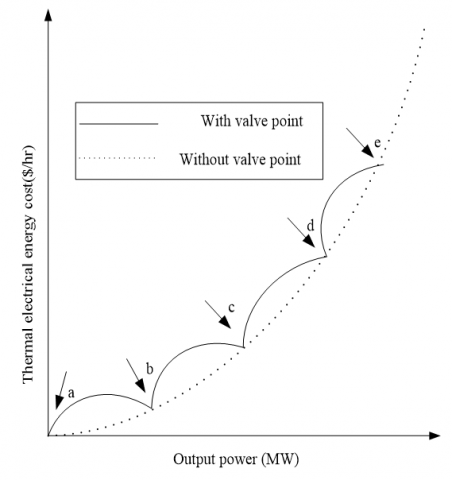Figure 1. Input and output curve with and without valve-point loading effect- a, b, c, d and e are valve points

Figure 1 shows the operating cost characteristics of thermal station generators with and without valve-points loading Effect.

Figure 2 illustrates the step by step procedure of TLBO algorithm to optimize the ELD problem in the power system network.

## 2.jpg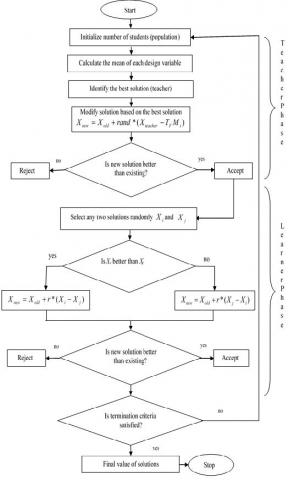Figure 2. TLBO algorithm flow chart

3.2 Test system1: Six unit test system

This case, a non-convex cost function based 6 thermal units are considered. The proposed method effectiveness is tested on two different load demands 800 and 1263 MW that can be meet by 6 thermal units. The test system data taken from Ref. . In this case population size is assumed as 60. The TLBO & OTLBO load dispatch results are formulated in Table 1. In this case, 25 independent trails have been made with 200 iterations per trail. Based on the performance, three different methods results are compared shown in below Table 1 & 2.

From the Table 1, at load demand of 800MW, the obtained minimum cost by Lambda iteration is 9528.7222\$/h with the power loss of 5.9642MW. The obtained minimum cost by OTLBO method is 9528.7969\$/h with the power loss of 5.9597 MW. The cost obtained by TLBO is 9528.8844\$/h with power loss of 6.0179 MW. From the records, its clearly shows that the obtained minimum cost by all the methods is almost same as the global solution at the load demand of 800 MW.From Table 2, now the power demand of 1263MW, the obtained minimum cost by Lambda iteration method and OTLBO is 15449.8995\$/h with the power loss of 12.9582MW. The minimum cost obtained by TLBO is 15450.6753\$/h with the power loss of 12.8536MW. The cost obtained by Lambda iteration method and OTLBO is same as the global solution. Figure 3 shows the comparison of convergence characteristics at different populations for different methods. As shown fig x axis represents iterations and y axis represents minimum cost in \$/hr.

3.3 Test system 2: 10-unit system

This case, a non-convex cost function based 10 thermal units are considered. The performance of the proposed methods was demonstrated at two different load demands and that load demands meet by ten thermal units are 1500 and 2000MW. The test data taken from . Here 100 population size is taken. The dispatch results of 10-unit system using the proposed methods are given in Table 2. For this test system, trails of 25 independent are made with 300 iterations/trail. Based on data obtained, the comparisons of six thermal units test by different methods are presented in Table 3 & 4.

## 3.jpg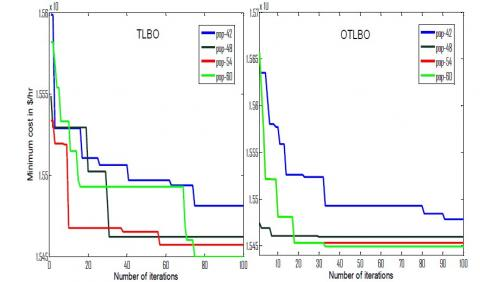Figure 3. Comparison of convergence characteristics for different populations

From the Table 3, at load demand of 1500MW the obtained minimum cost by Lambda iteration technique and TLBO is 81130.0325\$/h with the power loss of 49.0223MW. The obtained minimum cost by OTLBO technique is 81129.7603\$/h with the power loss of 49.007MW. From the above records it says clearly that the obtained minimum cost by the OTLBO is the global solution at the load demand of 1500MW.

Table 1. Comparisonal results for 6-unit system with load demand of 800 MW

 Unit PD=800 MW Lambda TLBO OTLBO 1 342.2421 343.4325 339.6431 2 95.4819 96.5919 96.5813 3 181.9937 183.1756 183.2407 4 53.6758 50 53.9589 5 82.5707 82.8179 82.5354 6 50.0000 50 50 Generation cost in \$/hr 9528.7222 9528.8844 9528.7969 Power loss in MW 5.9642 6.0179 5.9597 Table 2. Comparisonal results for 6-unit system with load demand of 1263 MW  Unit PD=1263 MW Lambda TLBO OTLBO 1 447.5038 444.4068 447.5038 2 173.3182 170.8177 173.3182 3 263.4628 263.9355 263.4628 4 139.0653 146.5230 139.0652 5 165.4734 166.4267 165.4733 6 87.1347 83.7436 87.1347 Generation cost in \$/hr 15449.8995 15450.6753 15449.8995 Power loss in MW 12.9582 12.8536 12.9582

Table 3. Comparisonal results for 10-unit system with demand of 1500 MW

 Unit PD=1500MW Lambda TLBO OTLBO 1 43.5706 43.5706 45.6086 2 60.8157 60.8157 61.7683 3 72.1301 72.1301 67.6629 4 60.3987 60.3987 55.5074 5 51.3367 51.3367 51.4848 6 71.3367 71.3367 71.4848 7 207.1676 207.1676 209.5246 8 222.2243 222.2243 232.5880 9 372.1789 372.1789 375.2049 10 387.8631 387.8631 378.1727 Generation cost in \$/hr 81130.0325 81130.0325 81129.7603 Power loss in MW 49.0223 49.0223 49.0070 From Table 4, now power demand of 2000MW the obtained minimum cost by Lambda iteration method is 111261.5057\$/h with the power loss of 87.0403MW. The obtained minimum cost by OTLBO method is 11261.5051\$/h with the power loss of 87.0403MW. The TLBO obtained cost is 111289.9482\$/h with power loss of 87.1252. Therefore, the cost obtained by Lambda iteration method and OTLBO is almost same but the cost obtained by OTLBO method is global minimum.

Figure 4 shows the graphical representation of comparison convergence characteristics of obtained minimum cost for 20runs at load demand 2000 MW. As shown in fig the cost obtained by Lambda iteration method is constant for all runs while the other methods are varying.

Table 4. Comparisonal results for 10-unit system with demand of 1500 MW

 Unit PD=2000 MW Lambda TLBO OTLBO 1 55.0000 55.0000 55.0000 2 80.0000 80.0000 80.0000 3 107.0165 120.0000 107.0151 4 99.9004 95.5547 99.9007 5 81.9005 77.8408 81.9024 6 83.2229 78.7297 83.2221 7 300.0000 300.0000 300.0000 8 340.0000 340.0000 340.0000 9 470.0000 470.0000 470.0000 10 470.0000 470.0000 470.0000 Generation cost in \$/hr 111261.5057 111289.9482 111261.5051 Power loss in MW 87.0403 87.1252 87.0403 ## 4.jpg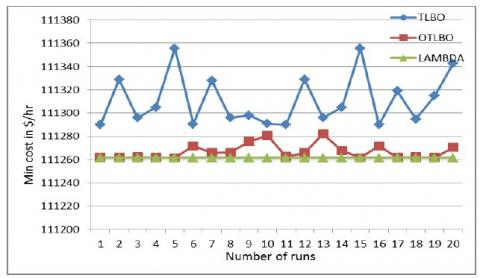Figure 4. Comparison of convergence characteristics of obtained minimum cost for 20runs 3.4 Test system 3: 14-unit system This case, a non-convex cost function based 14 thermal units are considered. The performance of the proposed methods is demonstrated at two different load demands and that load demands meet by 14 thermal units are 1500 and 2000MW. The data taken from Ref. . Here population is 140. The dispatch results of 14-unit system using the proposed methods are given in Tables 5 & 6. For this test system, 500 iterations per trail are made with 25 independent trails. From the data, six thermal units’ comparisons shown by different methods are presented in Tables 5 & 6. From the Table 5, power demand of 1500MW, obtained minimum cost by Lambda iteration method is 6612.5868 \$/h with the power loss of 17.9213MW. The obtained minimum cost by OTLBO technique is 6612.5089 \$/h with the power loss of 18.1087 MW. TLBO produced the cost of 6612.5120 \$/h with power loss of 18.1655 MW. It has been showed that the minimum cost obtained by all the methods is almost same but the cost obtained by OTLBO is the global solution at the load demand of 1500MW.

From Table 6, now at the power demand of 2000MW, the obtained cost from all the methods is almost same but the cost obtained by OTLBO method is global minimum and it is 8895.4566\$/h with power loss of 30.7713 MW. Table 5. Comparisonal results for 14-unit system with demand of 1500 MW  Unit PD=1500 MW Lambda TLBO OTLBO 1 221.3101 220.1858 218.5729 2 189.0354 192.2743 190.7673 3 50.5688 49.1485 53.1257 4 88.2294 86.0241 88.1582 5 150.0000 150.0258 150.0026 6 135.0000 135.0258 135.0026 7 135.0000 135.0258 135.0026 8 60.0000 60.0258 60.0026 9 139.6414 139.3569 136.2976 10 127.1018 130.8644 132.3087 11 79.9875 80.0000 80.0000 12 79.9875 80.0000 80.0000 13 47.0593 45.1827 43.8651 14 15.0000 15.0258 15.0026 Generation cost in \$/hr 6612.5868 6612.5120 6612.5089 Power loss in MW 17.9213 18.1655 18.1087

Table 6. Comparisonal results for 14-unit system with demand of 2000 MW

 Unit PD=2000 MW Lambda TLBO OTLBO 1 310.6826 308.1352 312.1435 2 269.8385 276.6890 271.6552 3 120.5517 117.5742 116.8470 4 129.9988 130.0000 130.0000 5 192.9272 192.3146 193.8548 6 163.3757 162.2258 165.2621 7 136.9125 136.0410 136.1677 8 84.6855 86.0736 82.8410 9 162.0000 162.0000 162.0000 10 159.9811 160.0000 160.0000 11 80.0000 80.0000 80.0000 12 80.0000 80.0000 80.0000 13 85.0000 85.0000 85.0000 14 55.0000 55.0000 55.0000 Generation cost in \\$/hr 8895.6328 8895.5806 8895.4566 Power loss in MW 30.9535 31.0534 30.7713

## 5.jpg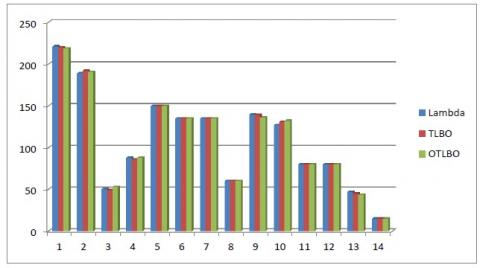Figure 5. Distribution of generation conceded by ten generators at PD=1500 MW

Figure 4 shows how the generation shared by fourteen generators with respect to their minimum and maximum limits which means it satisfies the inequality constraint. From the equality constraint, the fourteen generators generation should meet to given load demand.

4. Conclusion

In this paper, standard ELD problem can be solved in different cases with different methods. In first case ELD problem is represented with non-convex cost-function, already present there in network. The algorithms TLBO & OTLBO are successfully used to minimize the ELD problem considering 6, 10 and 14-unit test systems and also distinguished with lambda technique to test the performance of the proposed algorithm. The proposed algorithm OTLBO found better solution for all test systems than TLBO. This investigation results certainly says that the proposed method can be utilized as effective optimization providing better satisfactory solutions for ELD problems.  The paper established algorithms for the ELD problem to have the optimal solution for valve point loading effect only. However i strongly recommend that, in few cases there is still a need to investigate more avenues such as prohibited operating zones, ramp rate limits and multiple fuel selections for each unit. Here only thermal generating units have been considered. The ELD of hydro units can be applied by engaging these novel techniques. I also recommend that the new techniques have been used for combined hydrothermal economic load dispatch for future scope.

References

 Wollenberg, B.F., Wood, A.J., Sheble, G.B. (2013). Power Generation, Operation, and Control. 3rd Edition. Wiley Publication.

 Nasiri-Rad, H., Amjady, N. (2010). Solution of nonconvex and nonsmooth economic dispatch by a new Adaptive Real Coded Genetic Algorithm. Expert Systems with Applications, 37(7): 5239-5245. https://doi.org/10.1016/j.eswa.2009.12.084

 Rao, R.V., Patel, V. (2013). An improved teaching-learning-based optimization algorithm for solving unconstrained optimization problems. Scientia Iranica D, 20(3): 710-720. https://doi.org/10.1016/j.scient.2012.12.005

 Rajan, C.C.A. (2010). A solution to the economic dispatch using EP based SA algorithm on large scale power system. International Journal of Electrical Power & Energy Systems, 32(6): 583-591. https://doi.org/10.1016/j.ijepes.2009.11.014

 Barisal, A.K., Hota, P.K., Chakrabarti, R. (2010). Economic emission load dispatch through fuzzy based bacterial foraging algorithm. International Journal of Electrical Power & Energy Systems, 32(7): 794-803. https://doi.org/10.1016/j.ijepes.2010.01.016

 Ngamroo, I., Pothiya, S., Kongprawechnon, W. (2010). Ant colony optimisation foreconomic dispatch problem with non-smooth cost functions. International Journal of Electrical Power & Energy Systems, 32(5): 478-487. https://doi.org/10.1016/j.ijepes.2009.09.016

 Lin, W.M., Lee, J.C., Liao, G.C., Tsao, T.P. (2011). Quantum genetic algorithm for dynamic economic dispatch with valve-point effects and including wind power system. International Journal of Electrical Power & Energy Systems, 33(2): 189-197. https://doi.org/10.1016/j.ijepes.2010.08.014

 Mukherjee, V., Shaw, B., Ghoshal, S.P. (2011). Seeker optimization algorithm: application to the solution of economic load dispatch problems. IET Generation, Transmission & Distribution, 5(1): 81-91. https://doi.org/10.1049/iet-gtd.2010.0405

 Simon, S.P., Hemamalini, S. (2011). Dynamic economic dispatch using artificial immune system for units with valve-point effect. International Journal of Electrical Power & Energy Systems, 33(4): 868-874. https://doi.org/10.1016/j.ijepes.2010.12.017

 Sharma, D., Kumar, R., Sadu, A. (2011). A hybrid multi-agent based particle swarm optimization algorithm for economic power dispatch. International Journal of Electrical Power & Energy Systems, 33(1): 115-123. https://doi.org/10.1016/j.ijepes.2010.06.021

 Subrahmanyam, N., Chandram, K., Sydulu, M. (2011). Equal embedded algorithm for economic load dispatch problem with transmission losses. International Journal of Electrical Power & Energy Systems, 33(3): 500-507. https://doi.org/10.1016/j.ijepes.2010.12.002

 Mohammadi-Ivatloo, B., Rabiee, A., Moradi-Dalvand, M. (2014). Fast dynamic economic power dispatch problems solution via optimality condition decomposition. IEEE Transactions on Power Systems, 29(2): 982-985. https://doi.org/10.1109/TPWRS.2013.2288028

 Rashidi-Nejad, M., Pourakbari-Kasmaei, M. (2011). An effortless hybrid method to solve economic load dispatch problem in power systems. Energy Conversion and Management, 52(8-9): 2854-2860. https://doi.org/10.1016/j.enconman.2011.02.018

 Vijaya Muthu, P.S., Thanushkodi, K. (2011). An evolutionary programming based efficient particle swarm optimization for economic dispatch problem with valve-point loading. European Journal of Scientific Research, 52(3): 385-397. http://hdl.handle.net/10603/24146

 Panigrahi, B.K., Lohokare, M.R., Pattnaik, S.S. (2012). Neighborhood search-driven accelerated biogeography-based optimization for optimal load dispatch. IEEE Transactions on Systems, Man, and Cybernetics, Part C (Applications and Reviews), 42(5): 641-652. https://doi.org/10.1109/TSMCC.2012.2190401

 Imthias Ahamed, T.P., Jasmin, E.A., Jagathy Raj, V.P. (2011). Reinforcement learning approaches to economic dispatch problem. International Journal of Electrical Power & Energy Systems, 33(4): 836-845. https://doi.org/10.1016/j.ijepes.2010.12.008

 Ongsakul, W., Dieu, V.N., Polprasert, J. (2013). The augmented Lagrange Hopfield network for economic dispatch with multiple fuel options. Mathematical and Computer Modelling, 57(1-2): 30-39. https://doi.org/10.1016/j.mcm.2011.03.041

 Schegner, P., Dieu, V.N. (2013). Augmented Lagrange Hopfield network initialized by quadratic programming for economic dispatch with piecewise quadratic cost functions and prohibited zones. Applied Soft Computing, 13(1): 292-301. https://doi.org/10.1016/j.asoc.2012.08.026

 Singh, C., Wang, L. (2008). Stochastic economic emission load dispatch through a modified particle swarm optimization algorithm. Electric Power Systems Research, 78(8): 1466-1476. https://doi.org/10.1016/j.epsr.2008.01.012

 Lin, W.M., Gow, H.J., Tsai, M.T. (2011). A novel stochastic search method for the solution of economic dispatch problems with non-convex fuel cost functions. International Journal of Electrical Power & Energy Systems, 33(4): 1070-1076. https://doi.org/10.1016/j.ijepes.2011.01.026

 Savsani, V.J., Rao, R.V., Vakharia, D.P. (2011). Teaching-learning-based optimization: A novel method for constrained mechanical design optimization problems. Computer-Aided Design, 43(3): 303-315. https://doi.org/10.1016/j.cad.2010.12.015

 Pal, T., Roy, P.K., Pradhan, M. (2016). Grey wolf optimization applied to economic load dispatch problems. International Journal of Electrical Power & Energy Systems, 83: 325-334. https://doi.org/10.1016/j.ijepes.2016.04.034

 Simon, P., Hemamalini, S. (2008). Emission constrained economic dispatch with valve point effect using particle swarm optimization. IEEE International conference (TENCON 2008), 1-6. https://doi.org/10.1109/TENCON.2008.4766473

 Basu, M. (2011). Economic environmental dispatch using multi-objective differential evolution. Applied Soft Computing, 11(2): 2845-2853. https://doi.org/10.1016/j.asoc.2010.11.014

 Chakraborty, N., Mandal, K.K. (2008). Effect of control parameters on differential evolution based combined economic emission dispatch with valve-point loading and transmission loss. International Journal of Emerging Electric Power Systems, 9(4): 1-20. https://doi.org/10.2202/1553-779X.1918